# Performance of a real model: deconvolution of colorectal cancer samples

In Building new deconvolution models was shown the necessary workflow to build new deconvolution models. However, a ‘toy’ example was used in order to avoid long run times. In this vignette, the performance of a real model is shown in order to serve as a guide on how to assess whether a model has been trained correctly and can be used to deconvolute new bulk RNA-Seq samples.

The model to be shown has been built with data from Li et al. (2017) (GSE81861), so it can be used to deconvolute samples from colorectal cancer. It is loaded from digitalDLSorteRdata package and the corresponding trained model can be used through the digitalDLSorteRmodels package.

``````suppressMessages(library(ggplot2))
suppressMessages(library(digitalDLSorteR))
if (!requireNamespace("digitalDLSorteRdata", quietly = TRUE)) {
install.packages(
"digitalDLSorteRdata",
repos = "https://diegommcc.github.io/digitalDLSorteRdataRepo/"
)
}
suppressMessages(library(digitalDLSorteRdata))
data(DDLSLiComp.list)
DDLSLiComp <- listToDDLS(DDLSLiComp.list)
DDLSLiComp``````
``````## An object of class DigitalDLSorter
## Real single-cell profiles:
##   0 features and 0 cells
##   rownames: ---
##   colnames: ---
## Cell type composition matrices:
##   Cell type matrix for traindata: 13334 bulk samples and 10 cell types
##   Cell type matrix for testdata: 6666 bulk samples and 10 cell types
## Trained model: 30 epochs
##   Training metrics (last epoch):
##     loss: 0.0376
##     accuracy: 0.9265
##     mean_absolute_error: 0.0117
##     categorical_accuracy: 0.9265
##   Evaluation metrics on test data:
##     loss: 0.0614
##     accuracy: 0.9297
##     mean_absolute_error: 0.0141
##     categorical_accuracy: 0.9297
##   Performance evaluation over each sample: MAE MSE
## Project: DigitalDLSorterProject``````

As shown above, the model was trained 30 epochs with a total of 20,000 pseudo-bulk samples (13,334 for training and 6,666 for testing). Now, evaluation metrics will be calculated by the `calculateEvalMetrics` function in test data to explore its performance in depth.

``DDLSLiComp <- calculateEvalMetrics(DDLSLiComp)``

### How errors are distributed

With `distErrorPlot`, we can plot in different ways how errors are distributed. In this case, absolute error (`AbsErr`) by cell type (`CellType`) is shown. CD4 T cell proportions are predicted worse than the other cell types, but still have a median error close to zero.

``````distErrorPlot(
DDLSLiComp,
error = "AbsErr",
x.by = "CellType",
color.by = "CellType",
error.labels = FALSE,
type = "boxplot",
size.point = 0.5
)``````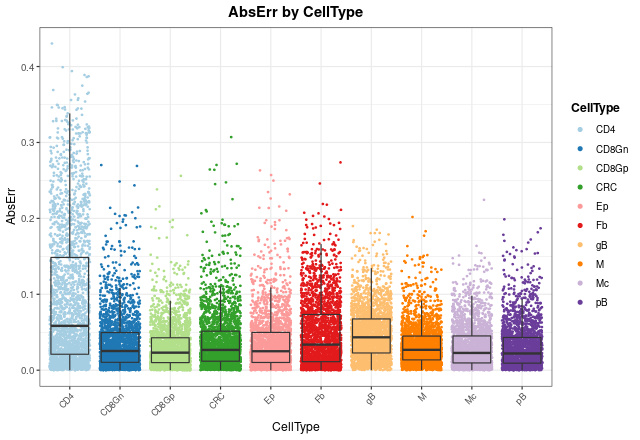In order to see mean error values, we can check it with `barErrorPlot`:

``barErrorPlot(DDLSLiComp, error = "MAE", by = "CellType")``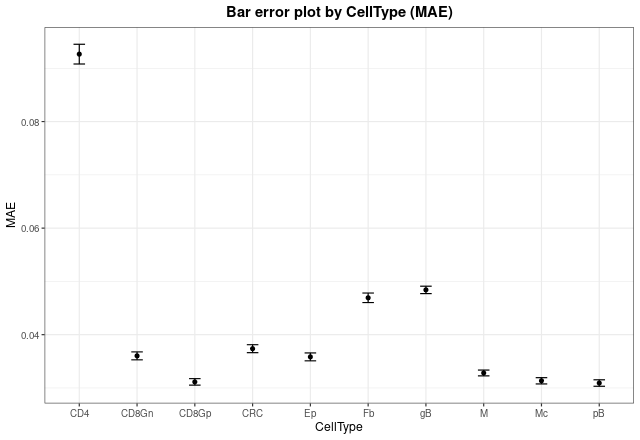Now, we can see in which proportions the model is failing most by changing the `x.by` argument to `"pBin"` and optionally setting `facet.by = "CellType"`:

``````distErrorPlot(
DDLSLiComp,
x.by = "pBin",
error = "AbsErr",
color.by = "CellType",
type = "boxplot",
size.point = 0.5
)``````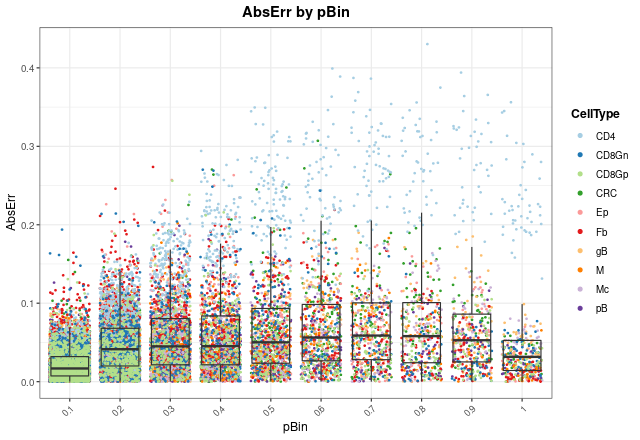``````distErrorPlot(
DDLSLiComp,
x.by = "pBin",
error = "AbsErr",
facet.by = "CellType",
color.by = "CellType",
error.label = TRUE,
type = "boxplot"
)``````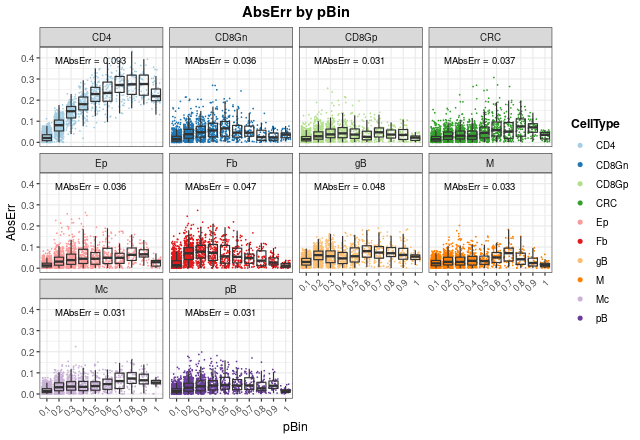As can be seen, most of the failures in CD4 T cell proportions occur at high proportions (between 0.5 and 1), and mean absolute errors (`MAbsErr`) shown as annotations in each panel are very low in all cases.

### Correlation between actual and expected proportions

Another way to visualize how the model works is to use the `corrExpPredPlot` function. Like `distErrorPlot`, we can see how proportions are correlated with each other by different variables. Here, we can see the same trend observed above: the model performs worst estimating CD4+ T cell proportions with the lowest concordance correlation and Pearson’s coefficients (\(R = 0.95\) and \(CCC = 0.794\), respectively). In fact, we can observe that the model tends to underestimate these proportions, although these values are still good. For the rest of cell types, the results show a very good performance with high coefficients (between 0.97 and 0.99).

``````corrExpPredPlot(
DDLSLiComp,
color.by = "CellType",
facet.by = "CellType",
corr = "both",
size.point = 0.5
)``````
``## `geom_smooth()` using formula 'y ~ x'``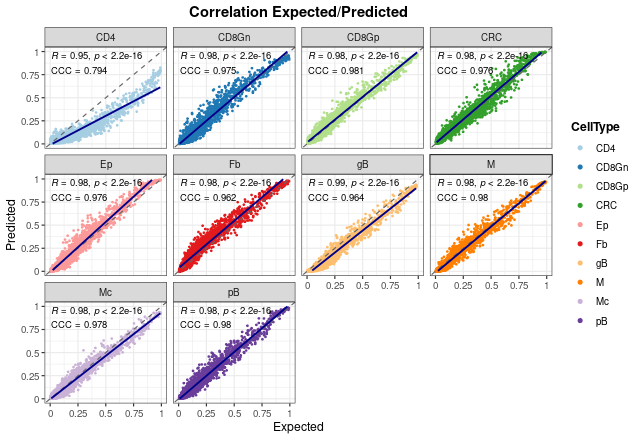It is important to note that these results only show the estimated proportions of pseudo-bulk samples. If we don’t filter out single-cell profiles, the results improve in general, showing that the model is able to deal with pure samples as well.

``````corrExpPredPlot(
DDLSLiComp,
color.by = "CellType",
facet.by = "CellType",
size.point = 0.5,
filter.sc = FALSE,
corr = "both"
)``````
``## `geom_smooth()` using formula 'y ~ x'``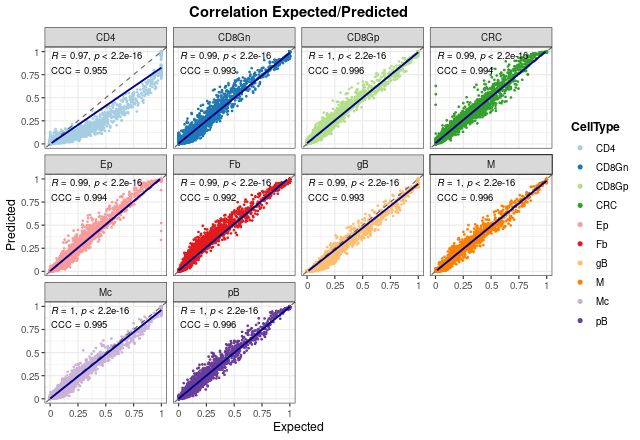In the end, we can see the overall results without splitting the graph. As can be seen, both coefficients present also high values when all proportions are considered.

``````corrExpPredPlot(
DDLSLiComp,
color.by = "CellType",
size.point = 0.5,
corr = "both"
)``````
``## `geom_smooth()` using formula 'y ~ x'``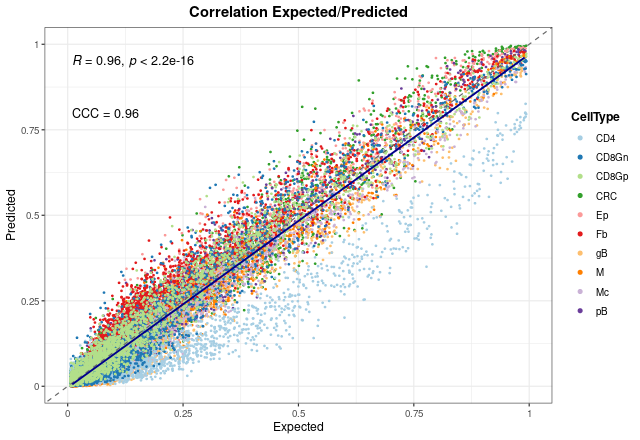### Bland-Altman agreement plots

The last way to graphically represent results is to use `blandAltmanLehPlot`. It generates a Bland-Altman agreement plot, a method for analyzing the level of agreement between two variables, in that case expected vs predicted cell proportions. As shown, most of the proportions fall close to zero (note blue density lines) and the dashed red lines are very close to the mean, although we can observe again the aforementioned problem in the estimate of T CD4 cell proportions.

``````blandAltmanLehPlot(
DDLSLiComp,
color.by = "CellType",
size.point = 0.5,
filter.sc = TRUE,
density = TRUE
)``````